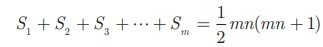Home | | Maths 10th Std | Exercise 2.6: Series: Sum to n terms of an A.P.(Arithmetic Progression)

# Exercise 2.6: Series: Sum to n terms of an A.P.(Arithmetic Progression)

Maths Book back answers and solution for Exercise questions - Mathematics : Numbers and Sequences: Series: Sum to n terms of an A.P.(Arithmetic Progression): Exercise Questions with Answer

Exercise 2.6

1. Find the sum of the following

(i) 3,7,11,… up to 40 terms.

(ii) 102,97,92,… up to 27 terms.

(iii) 6 + 13 + 20 +  + 972. How many consecutive odd integers beginning with 5 will sum to 480?3. Find the sum of first 28 terms of an A.P. whose nth term is 4n - 3 .4. The sum of first n terms of a certain series is given as 2n 2 - 3n . Show that the series is an A.P.5. The 104th term and 4th term of an A.P. are 125 and 0. Find the sum of first 35 terms.6. Find the sum of all odd positive integers less than 450.7. Find the sum of all natural numbers between 602 and 902 which are not divisible by 4.8. Raghu wish to buy a laptop. He can buy it by paying ₹40,000 cash or by giving it in 10 installments as ₹4800 in the first month, ₹4750 in the second month, ₹4700 in the third month and so on. If he pays the money in this fashion, find

(i) total amount paid in 10 installments.

(ii) how much extra amount that he has to pay than the cost?9. A man repays a loan of ₹65,000 by paying ₹400 in the first month and then increasing the payment by ₹300 every month. How long will it take for him to clear the loan?10. A brick staircase has a total of 30 steps. The bottom step requires 100 bricks. Each successive step requires two bricks less than the previous step.

(i) How many bricks are required for the top most step?

(ii) How many bricks are required to build the stair case?11. If S 1 , S2,S 3 , ...,Sm  are the sums of n terms of m A.P.’s whose first terms are 1, 2, 3,..., m and whose common differences are 1, 3, 5, ...,(2m -1)  respectively, then show that.12. Find the sum.1.(i) 3240 (ii) 999 (iii) 721

2. 20

3. 1540

5. 612.5

6.50625

7. 168448

8.(i) ₹ 45750 (ii) ₹ 5750

9. 20 months

10.(i) 42 (ii) 2130

12. 6/(a+b) / (24a - 13b)

Tags : Problem Questions with Answer, Solution | Mathematics , 10th Mathematics : UNIT 2 : Numbers and Sequences
Study Material, Lecturing Notes, Assignment, Reference, Wiki description explanation, brief detail
10th Mathematics : UNIT 2 : Numbers and Sequences : Exercise 2.6: Series: Sum to n terms of an A.P.(Arithmetic Progression) | Problem Questions with Answer, Solution | Mathematics